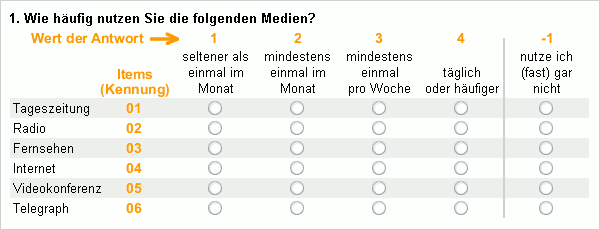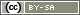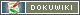# At Least One Item Selected

Sometimes, it is not sufficient for a filter to check individual answers; but rather all the items in a question have to be looked at. Whether it be to look up corresponding items in another question (Use Selected Items in Another Question) or just to check whether at least one item fulfils a particular condition. An exception to this is the calculation of scale indices within the survey (Count Points for Responses).

This chapter describes the function `getItems()` and loops – two tools which allow sophisticated filters over multiple items. The starting point of this chapter is the following question TF04: a scale. The scale's values and the item IDs are shown in the image for additional information.The task is now to check if the respondent uses at least one medium at least once a month. Of course, this could be done with 6 individual conditions linked using the AND operator (`and`) (Linking Multiple Conditions). How this can be achieved more efficiently and concisely is described below.

## Abstract

A list of selected items can be created with the function `getItems()`. Using PHP's own function `count()` the list can be checked to see if it contains at least one entry (i.e. an item).

The following PHP code assumes that question TF04 was asked on a previous page. The function `getItems()` lists all of the items that have got at least (`min`) a value of 2. If this list does not contain any entries (i.e. 0), the IF filter sends the participant to the end of the survey.

```// PHP code on page 2
\$selected = getItems('TF04', 'min', 2); // determine media used
if (count(\$selected) == 0) {
goToPage('end');  // go to the end, if none of the media are used
}```

The rest of the manual describes how the same problem can be resolved using a FOR loop. This procedure becomes interesting if the `getItems()` function is insufficient.

## Loops

Basically, all the items (No. 1 to 6) have to be checked in the same way. Loops are there to carry out these monotonous tasks. A FOR loop does nothing other than enumerate a number.

The following example demonstrating loops writes a line of text 6 times with slightly modified content in the questionnaire.

The loop saves the number, which increases by 1 in each pass, in the variable `\$i` (PHP Variables). In this example, the FOR counter begins with 1 (`\$i=1`). Then, as long as the number `\$i` is smaller or equal to 6 (`\$i<=6`) for that amount of time, the number in `\$i` increases by one (`\$i++`). All the numbers from 1 to 6 are passed through: whatever is behind the `for` in curly brackets is executed six times – each one with a different `\$i`.

```for (\$i=1; \$i<=6; \$i++) {
html((string)\$i);
}```

The above loop writes the number simply in the questionnaire – the result is trivial:

`123456`

What makes the whole thing more interesting is if an item ID is generated from the number. In the following example, the question ID is saved beforehand in the variable `\$question`. In the following loop, the text 'round', the number `\$i` and additional text fragments are combined with the period (`.`) and saved in the variable `\$text`. For example, the variable `\$text` then contains “round 1 with item TF04_01” in the first iteration.

```\$question = 'TF04';  // saves text 'TF04' in variable \$question
for (\$i=1; \$i<=6; \$i++) {
\$text = 'Round '.\$i.' with item '.\$question.'_0'.\$i; // combined
html('<p>'.\$text.'</p>');                           // write
}```

The PHP code generates the following output in the questionnaire, which already contain item IDs:

```Round 1 with item TF04_01
Round 2 with item TF04_02
Round 3 with item TF04_03
Round 4 with item TF04_04
Round 5 with item TF04_05
Round 6 with item TF04_06```

## Loops und Filters

Of course, not only the item IDs should be written. The following example checks which types of media are used at least once a month (at least an answer code of 2). In order to do this, the question TF04 has to have been asked on one of the previous pages. The ID of the item is created by linking the question ID and the number, saved in the variable `\$item` and subsequently used in `value()`.

The variable `\$counter` is set to 0 beforehand. The counter will increase by one with every medium used at least once a month (`\$counter++` is synonymous with `\$counter = \$counter + 1`).

```\$question = 'TF04'; // if the ID is needed more frequently
\$number_items = 6;  // 6 items are checked
\$counter = 0;       // sets the counter to 0
for (\$i=1; \$i<=\$number_items; \$i++) {
\$item = \$question.'_'.\$i;  // gives e.g. TF04_1
if (value(\$item) >= 2) {   // checks item TF01_1 to TF04_6
html('<p>Medium no. '.\$i.' used regularly</p>');
\$counter++;  // increases counter by one
}
}

// display information
html('<p>Used regularly '.\$counter.' media</p>');

// Filter
if (\$counter == 0) {
goToPage('end');  // go to the end if none of the media are used
}```

Tip: If the item ID is only stated as a single-digit ((`TF04_1` instead of `TF04_01`), the `value()` command is not affected. This is particularly useful if the user wants to check more than 9 items.

In this example, it is particularly interesting what can be done with the counter (`\$counter`) – i.e. the last three lines. If an respondent uses none of the specified media at least once a month, in the above example he will be sent directly to the end of the questionnaire. Of course, a question block could also be skipped in this way or other filters implemented depending on `\$counter`.

Tip: If you need the complex determined variable `\$counter` later on in the questionnaire for another filter, use the function `registerVariable()`.

It is often the case that only items for the media also used should be used in another question. This process is explained in the chapter Use Selected Items in Another Question.